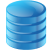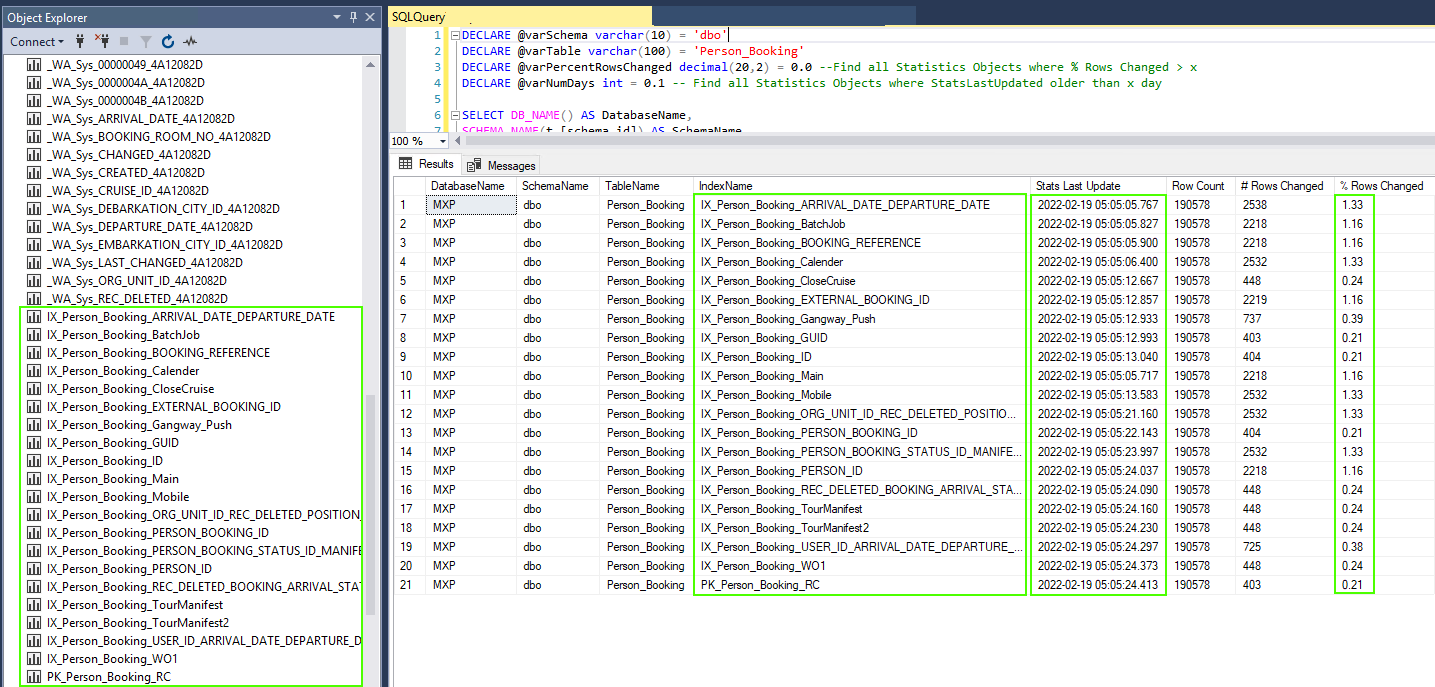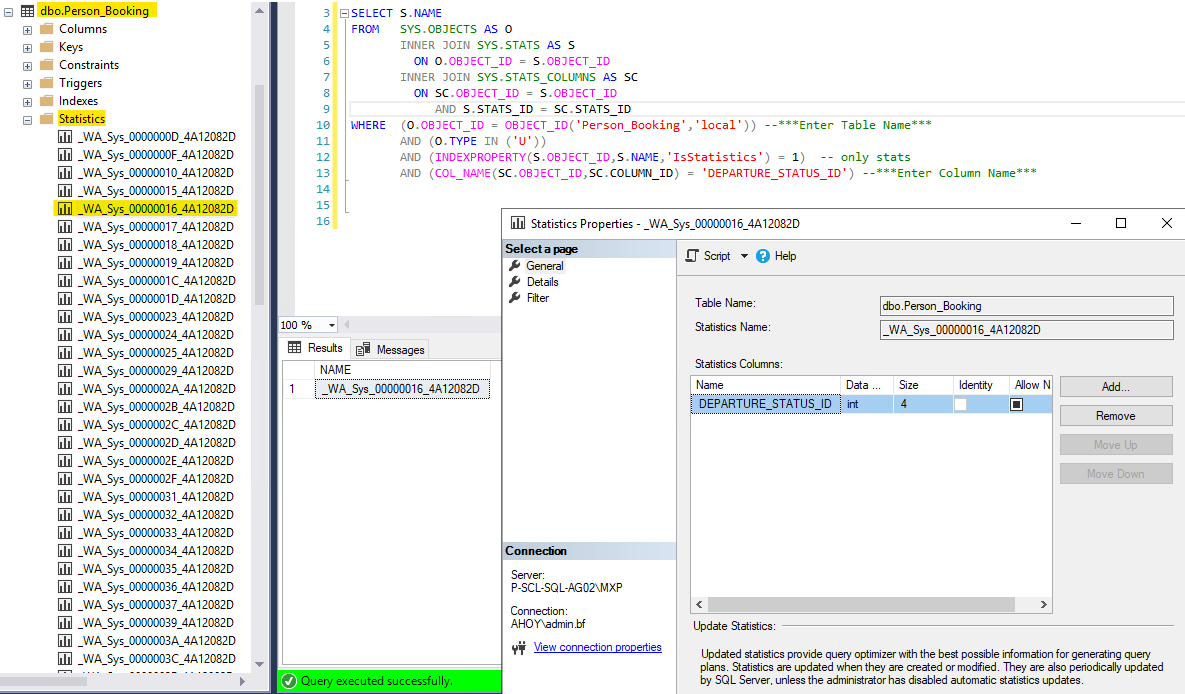info@techdevops.com | 437-991-3573 | Data Engineering ServicesTechDevOps.com
 Resources Tools Services
 Experts in Microsoft SQL Server on Windows, Linux, Containers | Clusters, Always On, FCI | Migrations, Cloud, Performance

Statistics Objects Information
by BF (Principal Consultant; Architecture; Engineering)
2022-02-16

 Statistics store data distribution.What are the different types of Statistics?There are two types of statistics: Column Statistics and Index Statistics. Column Statistics are statistics objects linked to columns and created automatically & SQL Server creates these unless AUTO_CREATE_STATISTICS is disabled.Index Statistics are statistics linked/corresponding to indexes and these statistics are created at the same time when the indexes are. Also, Index Statistics are updated with FULLSCAN when indexes are being rebuilt. Thus, there is no need to update Index Statistics after rebuilding the indexes.(If one did, it might be counter-productive if using UPDATE STATISTICS command without parameters as that is based on a sampling only)When to create statistics?The Query Optimizer already creates statistics in the following ways:1. The Query Optimizer creates statistics for indexes on tables or views when the index is created. These statistics are created on the key columns of the index. (Indexes have a list of Key Columns and then another list of Included Columns)If the index is a filtered index, the Query Optimizer creates filtered statistics on the same subset of rows specified for the filtered index. 2. The Query Optimizer creates statistics for single columns in query predicates when AUTO_CREATE_STATISTICS is on.Each statistics object is created on a list of one or more table columns and includes a histogram displaying the distribution of values. The actual Histogram is always based only on the first column of the index even if the index is composite one. This is one of the reason why it is always suggested to have most selective column as the first column of the index, but there are exceptions. Note: Statistics objects on multiple columns also store statistical information about the correlation of values among the columns. These correlation statistics, or densities, are derived from the number of distinct rows of column values.For most queries, these two methods for creating statistics ensure a high-quality query plan; in a few cases, you can improve query plans by creating additional statistics with the CREATE STATISTICS statement. These additional statistics can capture statistical correlations that the Query Optimizer does not account for when it creates statistics for indexes or single columns. Your application might have additional statistical correlations in the table data that, if calculated into a statistics object, could enable the Query Optimizer to improve query plans.(Link: https://docs.microsoft.com/en-us/sql/relational-databases/statistics/statistics?view=sql-server-ver15)Note: If statistics correspond to an index, the stats_id value in the sys.stats catalog view is the same as the index_id value in the sys.indexes catalog view, and the following query returns the same results as the preceding query. If statistics do not correspond to an index, they are in the sys.stats results but not in the sys.indexes results.(Link: https://docs.microsoft.com/en-us/sql/t-sql/functions/stats-date-transact-sql?view=sql-server-ver15)Why are Statistics Objects so important?A SQL Server Query Plan, created by the Query Optimizer, is a set of instructions that describe how a query will be executed by the database engine. These Plans require various inputs & Statistics is one of those very important inputs. Statistics store the distribution of the column data of the tables in a histogram. This data is used to estimate how many rows will be returned by the plan operators and, based on those statistics, estimations are then calculated for the amount I/O, CPU & Memory system resources needed by the database engine.Statistics Objects linked/corresponding to Indexes: DECLARE @varSchema varchar(10) = 'dbo'DECLARE @varTable varchar(100) = ''DECLARE @varPercentRowsChanged decimal(20,2) = 0.0 --Find all Statistics Objects where % Rows Changed > xDECLARE @varNumDays int = 0.1 -- Find all Statistics Objects where StatsLastUpdated older than x daySELECT DB_NAME() AS DatabaseName,SCHEMA_NAME(t.[schema_id]) AS SchemaName,t.name AS TableName,ix.name AS IndexName,STATS_DATE(ix.id,ix.indid) AS 'Stats Last Update', -- using STATS_DATE functionix.rowcnt AS 'Row Count',ix.rowmodctr AS '# Rows Changed',CAST((CAST(ix.rowmodctr AS DECIMAL(20,8))/CAST(ix.rowcnt AS DECIMAL(20,2)) * 100.0) AS DECIMAL(20,2)) AS '% Rows Changed'FROM sys.sysindexes ixINNER JOIN sys.tables t ON t.[object_id] = ix.[id]WHERE ix.id > 100 -- excluding system object statisticsAND ix.indid > 0 -- excluding heaps or tables that do not any indexesAND ix.rowcnt >= 10000 -- only indexes with more than 10000 rowsAND (@varSchema = '' or SCHEMA_NAME(t.[schema_id]) = @varSchema)AND (@varTable = '' or t.name = @varTable)AND CAST((CAST(ix.rowmodctr AS DECIMAL(20,8))/CAST(ix.rowcnt AS DECIMAL(20,2)) * 100.0) AS DECIMAL(20,2)) >= @varPercentRowsChangedAND STATS_DATE(ix.id,ix.indid) < GetDate() - @varNumDays ORDER BY '% Rows Changed' DESC--ix.name ASCStatistics Objects linked/corresponding to Columns: SELECT S.NAMEFROM SYS.OBJECTS AS O INNER JOIN SYS.STATS AS S ON O.OBJECT_ID = S.OBJECT_ID INNER JOIN SYS.STATS_COLUMNS AS SC ON SC.OBJECT_ID = S.OBJECT_ID AND S.STATS_ID = SC.STATS_IDWHERE (O.OBJECT_ID = OBJECT_ID('Person_Booking','local')) --***Enter Table Name*** AND (O.TYPE IN ('U')) AND (INDEXPROPERTY(S.OBJECT_ID,S.NAME,'IsStatistics') = 1) -- only stats AND (COL_NAME(SC.OBJECT_ID,SC.COLUMN_ID) = 'DEPARTURE_STATUS_ID') --***Enter Column Name***